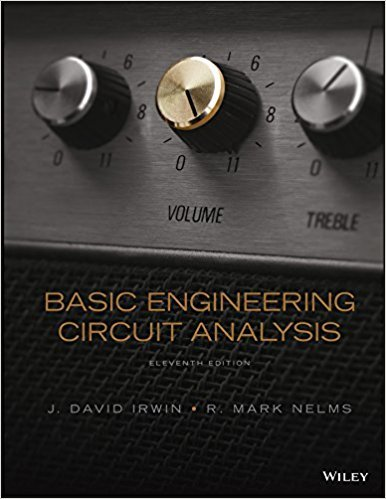×
Get Full Access to Basic Engineering Circuit Analysis - 11 Edition - Chapter 6 - Problem 6.35
Get Full Access to Basic Engineering Circuit Analysis - 11 Edition - Chapter 6 - Problem 6.35

×

# The current in a 4-mH inductor is given by the waveform inISBN: 9781118539293 159

## Solution for problem 6.35 Chapter 6

Basic Engineering Circuit Analysis | 11th Edition

• Textbook Solutions
• 2901 Step-by-step solutions solved by professors and subject experts
• Get 24/7 help from StudySoup virtual teaching assistantsBasic Engineering Circuit Analysis | 11th Edition

4 5 1 250 Reviews
10
4
Problem 6.35

The current in a 4-mH inductor is given by the waveform in Fig. P6.35. Plot the voltage across the inductor. 0.5 1.0 0.12 i(t) (mA) t (ms) Figure P6.35

Step-by-Step Solution:
Step 1 of 3

Tommy Gallant, Kevin Meyer, Hayden Brown ENGR 350-1 Dr. Stone 9/21/2016 Electronic Screw­Driver Report #2 9/21/2016 By; Kevin Meyer, Tommy Gallant, Hayden Brown Tommy Gallant, Kevin Meyer, Hayden Brown ENGR 350-1 Dr. Stone 9/21/2016 ENGR 350 Report 2 Executive Summary:

Step 2 of 3

Step 3 of 3

##### ISBN: 9781118539293

This textbook survival guide was created for the textbook: Basic Engineering Circuit Analysis, edition: 11. Basic Engineering Circuit Analysis was written by and is associated to the ISBN: 9781118539293. Since the solution to 6.35 from 6 chapter was answered, more than 255 students have viewed the full step-by-step answer. The full step-by-step solution to problem: 6.35 from chapter: 6 was answered by , our top Engineering and Tech solution expert on 11/23/17, 05:00AM. This full solution covers the following key subjects: inductor, given, fig, figure, current. This expansive textbook survival guide covers 15 chapters, and 1430 solutions. The answer to “The current in a 4-mH inductor is given by the waveform in Fig. P6.35. Plot the voltage across the inductor. 0.5 1.0 0.12 i(t) (mA) t (ms) Figure P6.35” is broken down into a number of easy to follow steps, and 29 words.

Unlock Textbook Solution